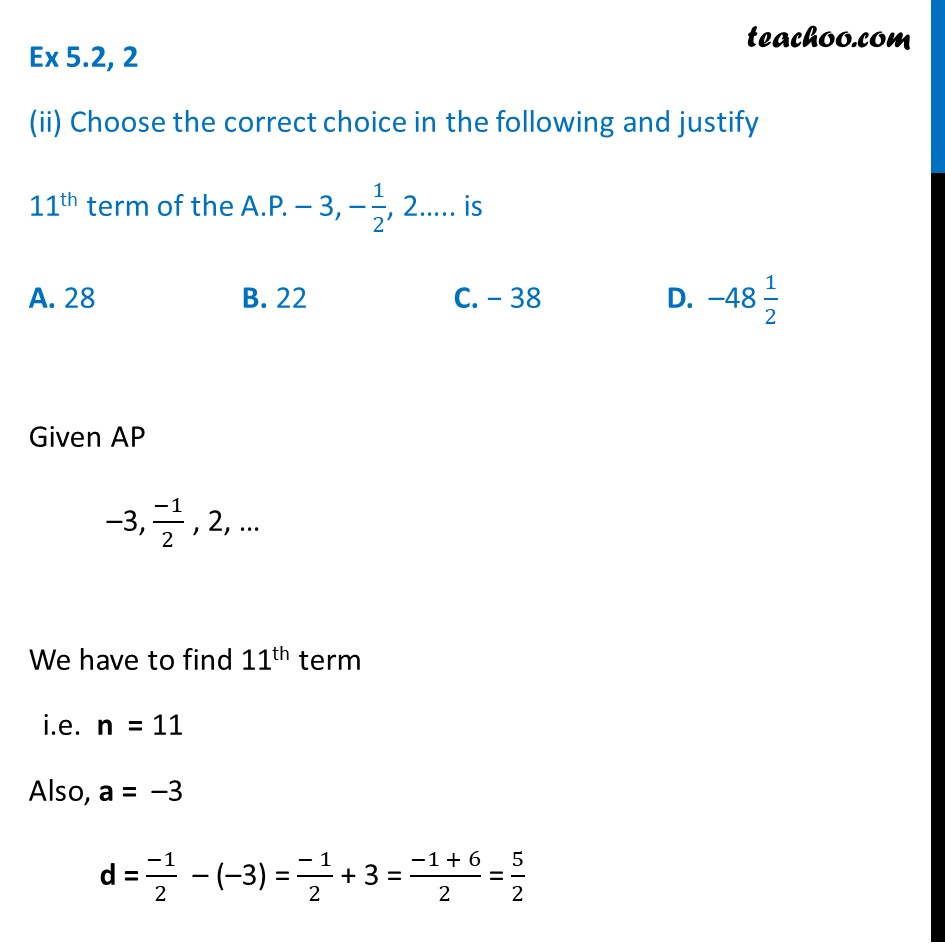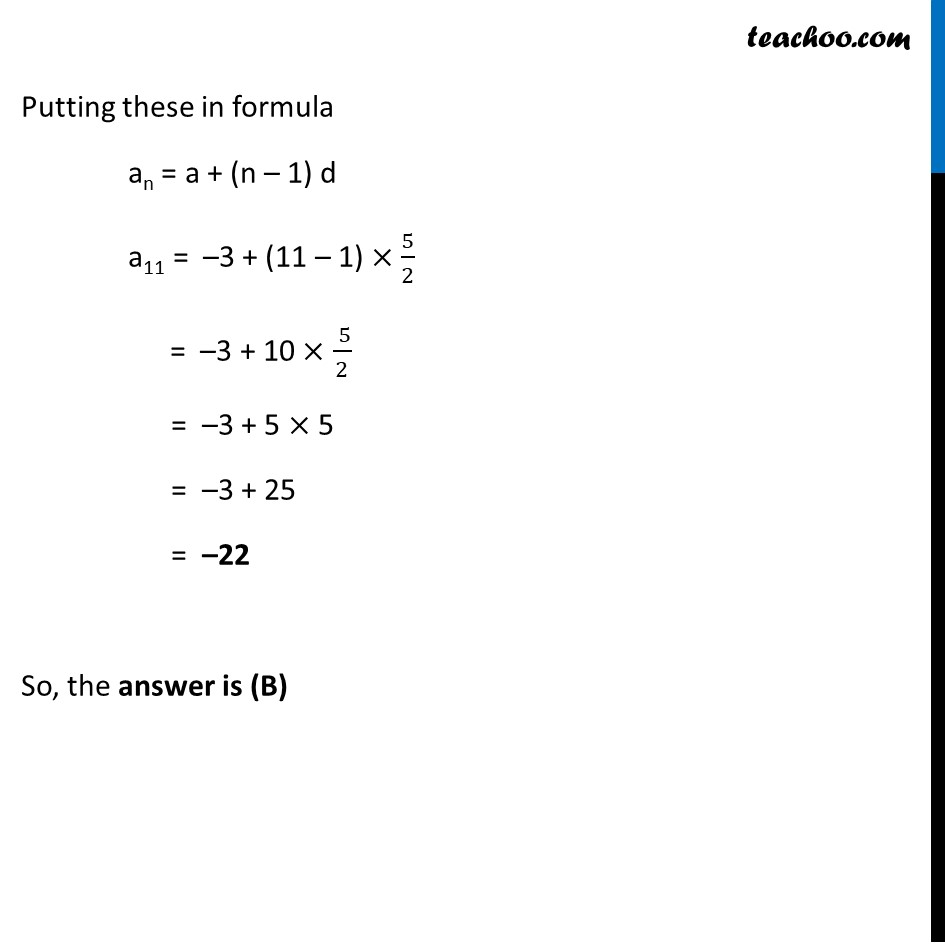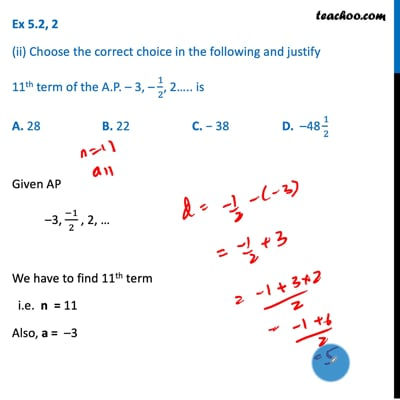Ex 5.2

Chapter 5 Class 10 Arithmetic Progressions
Serial order wiseThis video is only available for Teachoo black users

Maths Crash Course - Live lectures + all videos + Real time Doubt solving!

### Transcript

Ex 5.2, 2 (ii) Choose the correct choice in the following and justify 11th term of the A.P. – 3, – 1/2, 2….. is A. 28 B. 22 C. − 38 D. –48 1/2 Given AP –3, (−1)/2 , 2, … We have to find 11th term i.e. n = 11 Also, a = –3 d = (−1)/2 – (–3) = (− 1)/2 + 3 = (−1 + 6)/2 = 5/2 Putting these in formula an = a + (n – 1) d a11 = –3 + (11 – 1) × 5/2 = –3 + 10 × ( 5)/2 = –3 + 5 × 5 = –3 + 25 = –22 So, the answer is (B)